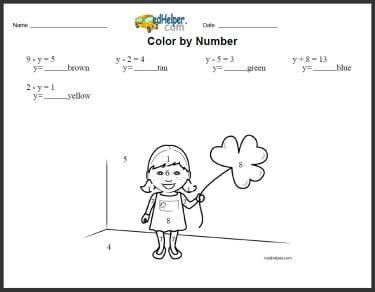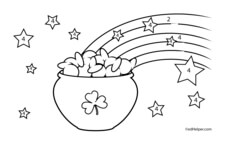Worksheets and No Prep Teaching Resources MathColor by Algebra

Math Lesson: Color by Numbers - February Color by Number BookAlgebra Coloring: solve the addition or subtraction equation

Math Lesson: Color by Numbers - March Color by Number BookAlgebra Coloring: solve the addition or subtraction equation

Math Lesson: Color by Numbers - August Color by Number BookAlgebra Coloring: solve the addition or subtraction equation

Have a suggestion or would like to leave feedback?